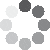Enter parameters Slabs, Footings & Walls
Enter parameters
Length:
ft
• inches (in)
• feet (ft)
• yards (yd)
• centimeters (cm)
• meters (m)
Width:
ft
• inches (in)
• feet (ft)
• yards (yd)
• centimeters (cm)
• meters (m)
Thickness:
in
• inches (in)
• feet (ft)
• yards (yd)
• centimeters (cm)
• meters (m)
Quantity:
Price per unit of volume:
yd³
• cubic yards (yd³)
• cubic feet (ft³)
• cubic inches (in³)
• cubic centimeters (cm³)
• cubic meters ()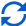Result
Volume:
0
yd³
• cubic yards (yd³)
• cubic feet (ft³)
• cubic inches (in³)
• cubic centimeters (cm³)
• cubic meters ()
Weight:
0
lb
• pounds (lb)
• US short tons(t)
• imperial-long tons (long t)
• kilograms (kg)
Bags:
0
40 lb
• 40 lb bags
• 50 lb bags
• 60 lb bags
• 80 lb bags
• 30 kg bags
• 50 kg bags
Сost:
$Enter parameters Diameter: in • inches (in) • feet (ft) • yards (yd) • centimeters (cm) • meters (m) Depth (Height): in • inches (in) • feet (ft) • yards (yd) • centimeters (cm) • meters (m) Quantity: Price per unit of volume: yd³ • cubic yards (yd³) • cubic feet (ft³) • cubic inches (in³) • cubic centimeters (cm³) • cubic meters ()Result Volume: 0 yd³ • cubic yards (yd³) • cubic feet (ft³) • cubic inches (in³) • cubic centimeters (cm³) • cubic meters () Weight: 0 lb • pounds (lb) • US short tons(t) • imperial-long tons (long t) • kilograms (kg) Bags: 0 40 lb • 40 lb bags • 50 lb bags • 60 lb bags • 80 lb bags • 30 kg bags • 50 kg bags Сost:$
Enter parameters
Outer Diameter:
ft
• inches (in)
• feet (ft)
• yards (yd)
• centimeters (cm)
• meters (m)
Inner Diameter:
ft
• inches (in)
• feet (ft)
• yards (yd)
• centimeters (cm)
• meters (m)
Length (Height):
in
• inches (in)
• feet (ft)
• yards (yd)
• centimeters (cm)
• meters (m)
Quantity:
Price per unit of volume:
yd³
• cubic yards (yd³)
• cubic feet (ft³)
• cubic inches (in³)
• cubic centimeters (cm³)
• cubic meters ()Result
Volume:
0
yd³
• cubic yards (yd³)
• cubic feet (ft³)
• cubic inches (in³)
• cubic centimeters (cm³)
• cubic meters ()
Weight:
0
lb
• pounds (lb)
• US short tons(t)
• imperial-long tons (long t)
• kilograms (kg)
Bags:
0
40 lb
• 40 lb bags
• 50 lb bags
• 60 lb bags
• 80 lb bags
• 30 kg bags
• 50 kg bags
Сost:
$Enter parameters Curb Depth: in • inches (in) • feet (ft) • yards (yd) • centimeters (cm) • meters (m) Curb Height: in • inches (in) • feet (ft) • yards (yd) • centimeters (cm) • meters (m) Gutter Width: in • inches (in) • feet (ft) • yards (yd) • centimeters (cm) • meters (m) Flag Thickness: in • inches (in) • feet (ft) • yards (yd) • centimeters (cm) • meters (m) Length: ft • inches (in) • feet (ft) • yards (yd) • centimeters (cm) • meters (m) Quantity: Price per unit of volume: yd³ • cubic yards (yd³) • cubic feet (ft³) • cubic inches (in³) • cubic centimeters (cm³) • cubic meters ()Result Volume: 0 yd³ • cubic yards (yd³) • cubic feet (ft³) • cubic inches (in³) • cubic centimeters (cm³) • cubic meters () Weight: 0 lb • pounds (lb) • US short tons(t) • imperial-long tons (long t) • kilograms (kg) Bags: 0 40 lb • 40 lb bags • 50 lb bags • 60 lb bags • 80 lb bags • 30 kg bags • 50 kg bags Сost:$
Enter parameters
Run:
in
• inches (in)
• feet (ft)
• yards (yd)
• centimeters (cm)
• meters (m)
Rise:
in
• inches (in)
• feet (ft)
• yards (yd)
• centimeters (cm)
• meters (m)
Platform Depth:
in
• inches (in)
• feet (ft)
• yards (yd)
• centimeters (cm)
• meters (m)
Width:
ft
• inches (in)
• feet (ft)
• yards (yd)
• centimeters (cm)
• meters (m)
Number of Steps:
Quantity:
Price per unit of volume:
yd³
• cubic yards (yd³)
• cubic feet (ft³)
• cubic inches (in³)
• cubic centimeters (cm³)
• cubic meters ()Result
Volume:
0
yd³
• cubic yards (yd³)
• cubic feet (ft³)
• cubic inches (in³)
• cubic centimeters (cm³)
• cubic meters ()
Weight:
0
lb
• pounds (lb)
• US short tons(t)
• imperial-long tons (long t)
• kilograms (kg)
Bags:
0
40 lb
• 40 lb bags
• 50 lb bags
• 60 lb bags
• 80 lb bags
• 30 kg bags
• 50 kg bags
Сost:
$Enter parameters Volume: yd³ • cubic yards (yd³) • cubic feet (ft³) • cubic inches (in³) • cubic centimeters (cm³) • cubic meters () Quantity: Price per unit of volume: yd³ • cubic yards (yd³) • cubic feet (ft³) • cubic inches (in³) • cubic centimeters (cm³) • cubic meters ()Result Weight: 0 lb • pounds (lb) • US short tons(t) • imperial-long tons (long t) • kilograms (kg) Bags: 0 40 lb • 40 lb bags • 50 lb bags • 60 lb bags • 80 lb bags • 30 kg bags • 50 kg bags Сost:$(9 votes, average: 4.56 out of 5)Loading...
Similar Calculators:
Real-time graphics. Make the calculations and see the changes.
Real-time graphics. Make the calculations and see the changes.
Real-time graphics. Make the calculations and see the changes.
Real-time graphics. Make the calculations and see the changes.
Real-time graphics. Make the calculations and see the changes.
Real-time graphics. Make the calculations and see the changes.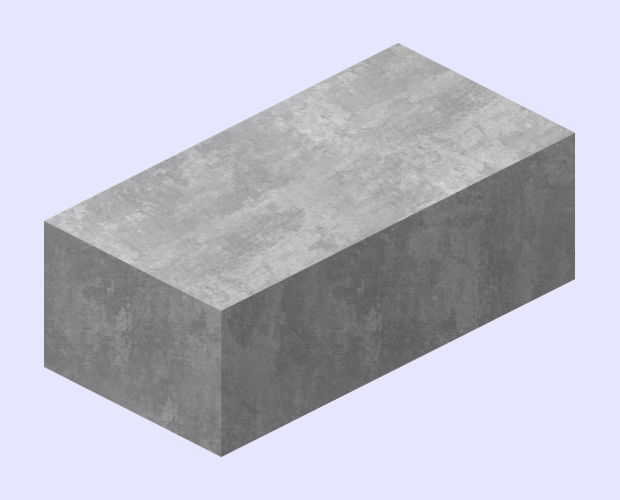Embed
Similar Calculators:When you start any construction project, it’s important that you have an idea of how much material you are likely to need.

We know this can be tricky when working with concrete, especially when estimating.

However, it’s easy to work this out by using our concrete calculator.

Not only will our calculator tell you how much concrete is required, it will also work out how many bags you will need and the total cost of the project.

Combined with a little preparation, our calculator can ensure you are ready for your next landscaping project! 👍

Contents:

## Concrete calculator formula

Using the measurements provided, our calculator first works out the volume of the required concrete mix using the formulae given below.

### Slabs, Footings and Walls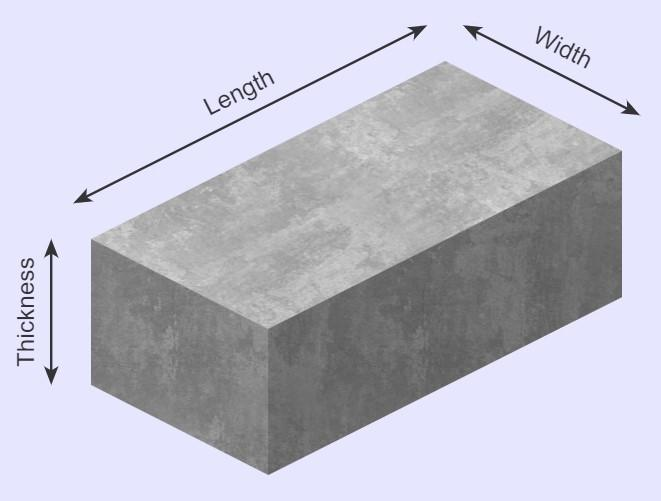The total volume is calculated using the formula:

$$Volume = Length \times Width \times Thickness$$

### Holes & Columns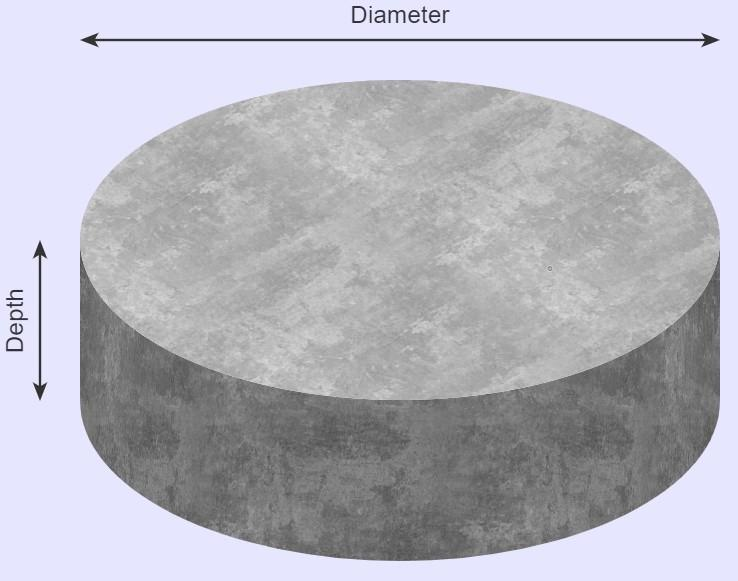The total volume is calculated using the formula:

$$Volume = \pi \times Radius^2 \times Depth$$

Where

$$Radius = {Diameter \over 2}$$

### Circular Slabs and Tubes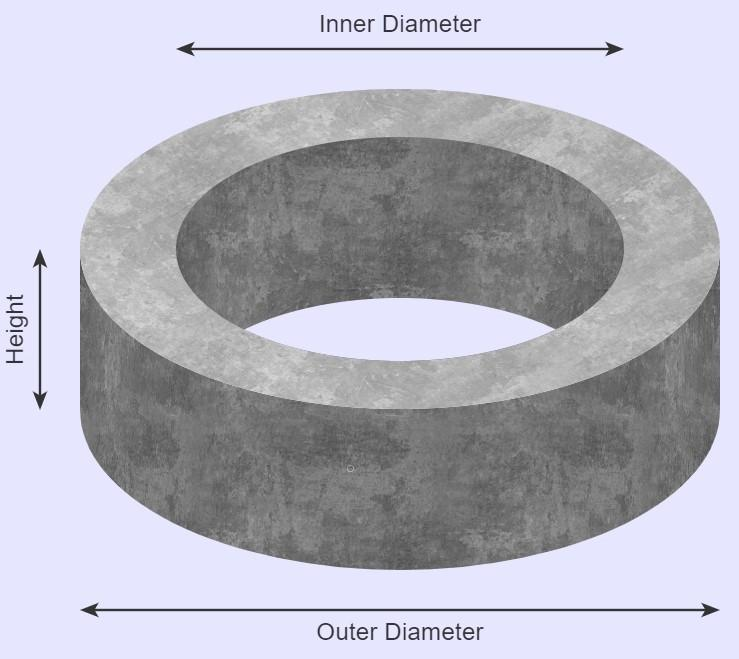The total volume is calculated using the formula:

$$Volume = \pi \times (Outer\,radius^2 – Inner\,radius^2) \times Height$$

Where

$$Radius = {Diameter \over 2}$$

### Curb & Gutter Barrier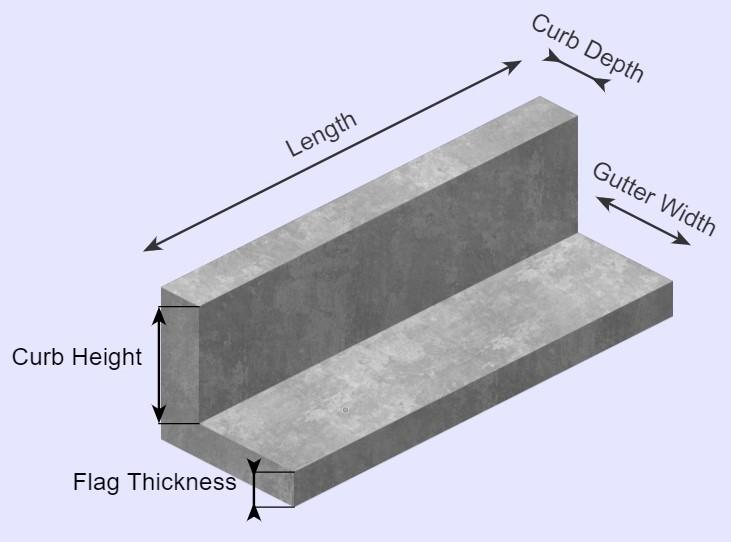The total volume is calculated using the formula:

$$Volume = Curb\,volume + Gutter\,volume$$

Where

$$Curb\,volume = Curb\,depth \times Curb\,height \times Length$$

and

$$Gutter\,volume = (Gutter\,width + Curb\,depth) \times Flag\,thickness \times Length$$

### Stairs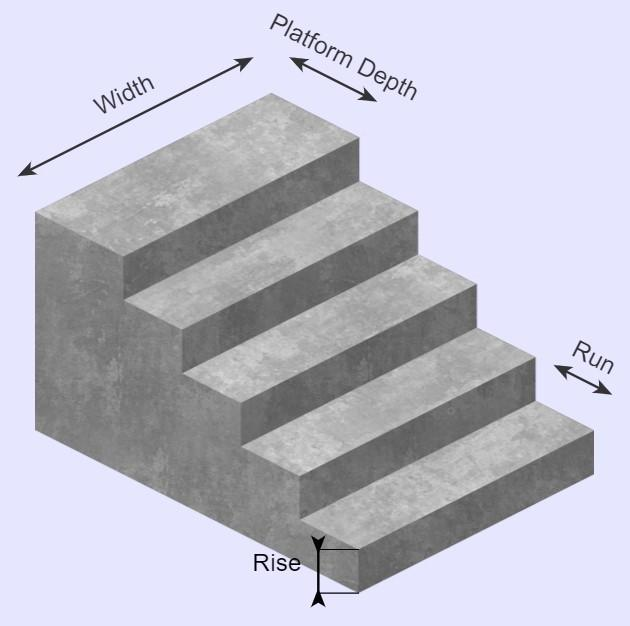The total volume is calculated using the formula:

$$Volume = Volume\,under\,platform + Volume\,under\,steps$$

$$Volume\,under\,platform = Platform\,depth \times Rise × Width \times Number\,of\,steps$$

$$Volume\,under\,step = Run \times Rise \times Width \times Number\,of\,Step$$

Note ⚠️

If there are multiple steps, separately calculate the volume under the steps for each and then sum to find the total volume under the steps (more details in the example).

Want to know the best part?

If you need to construct multiple concrete slabs/columns, etc., then our calculator can work out the total volume required:

$$Total\,volume = Volume \times Quantity$$

Our calculator also works out the total concrete mix weight and required number of bags (using a standard 40lb bag of concrete which weights 145 pounds per cubic foot):

$$Weight = Total\,volume \times 145\,lb/ft^3$$

$$Bags = {Weight \over 40\,lb}$$

Also, if you know the price per unit volume, then our calculator can work out the total cost:

$$Cost = Total\,volume \times Price\,per\,unit\,of\,volume$$

## But what happens if I’m measuring in different units?

It’s likely that you are wondering what to do if your units aren’t in feet and pounds.

Well, the solution couldn’t be easier!

Our calculator can do all the conversions for you, meaning you don’t have to.

For each measurement of length, you are able to work in metric units (feet, yards, inches) or imperial units (centimeters, meters).

Weight can be given in pounds (lb), US short tons (t), imperial-long tons (long t) or kilograms.

Simply select the desired units in the drop down options and our concrete calculator will do the following conversions:

$$1\,ft = 12\,in = 0.33\,yd = 30.48\,cm = 0.3048\,m$$

$$1\,t = 2000\,lb = 0.893\,long\,ton = 907\,kg$$

$$1\,ft^3 = 1728\,in^3 =0.037\,yd^3 = 28,317\,cm^3= 0.0283\,m^3$$

$$145\,lb/ft^3 = 2400\,kg/m^3 = 3915\,lb/yd^3$$

## Example calculations

Before we begin please note: The number of bags required must be rounded up, otherwise you’ll be left without sufficient concrete.

You’ll notice that we do this with all our examples (but the calculator does this for you!).

### Standard wall slab

Let’s say I need to construct a square concrete slab that is 12 feet wide (an area of 144 square feet) and 4 inches deep.

I want to calculate how many 40 lb bags of concrete I need.

The calculator would perform the following calculations to calculate that the slab would need 174 bags:

$$Volume = Length \times Width \times Thickness = 12\,ft \times 12\,ft \times 4\,in = 48\,ft^3$$

$$Weight = Total\,volume \times 145\,lb/ft^3 = 48\,ft^3 \times 145 = 6960\,lb$$

$$Bags = {Weight \over 40\,lb} = {6960\,lb \over 40\,lb} = 174\,bags$$

### 5 columns

Let’s say I need to construct 5 columns measuring 2 feet in diameter and 3 inches high. I want to estimate how many 40lb bags of concrete I need.

The gravel estimator does the following calculations:

$$Volume = \pi \times Radius^2 \times Depth = \pi \times 1\,ft^2 \times 3\,in = 0.7854\,ft^3$$

$$Total\,volume = Volume \times Quantity = 0.7854\,ft^3 \times 5 = 3927\,ft^3$$

$$Weight = Total\,volume \times 145\,lb/ft^3 = 3927\,ft^3 \times 145 = 569\,lb$$

$$Bags = {Weight \over 40\,lb} = {569\,lb \over 40\,lb} = 14.2 \rightarrow 15\,bags$$

### Circular slab with price per unit of volume

I want to construct a circular slab of concrete measuring 5 ft high.

I want to figure out how many bags of 40 lb concrete I need to do this.

The outer diameter is 10 ft and the inner diameter is 9 ft. The price of the concrete is \$108 per cubic yard.

The ready mix concrete calculator does the following calculations:

$$Volume = \pi \times (Outer\,radius^2 – Inner\,radius^2) \times Height = \pi \times (5\,ft^2 – 4.5\,ft^2) \times 5\,ft = 74.6\,ft^3$$

$$Weight = Volume \times 145\,lb/ft^3 = 74.6\,ft^3 \times 145 = 10819\,lb$$

$$Bags = {Weight \over 40\,lb} = {10819\,lb \over 40\,lb} = 270.4 \rightarrow 271\,bags$$

$$Cost = Volume \times Price\,per\,unit\,of\,volume = 74.6\,ft^3 \times 108\,/yd^3 = 298.45$$

But wait, there’s more!

We imagine you are wondering what happens if you want to use concrete bags weighing different amounts. 🤔

Well, our calculator can figure this out for you as well.

Simply select from the multiple options in the drop down menu to select a different weight.

### 4 curbs with price per unit volume and 50 lb bags of concrete

For our next example, let’s say we need to construct 4 curbs with the following measurements:

$$Curb\,Depth = 3\,in$$

$$Curb\,Height = 4\,in$$

$$Gutter\,Width = 10\,in$$

$$Flag\,Thickness = 3\,in$$

$$Length = 10\,ft$$

The calculator would first work out the total volume of the 4 curbs:

$$Curb\,volume = Curb\,depth \times Curb\,height \times Length = 3\,in \times 4\,in \times 10\,ft = 0.8333\,ft^3$$

$$Gutter\,volume = (Gutter\,width + Curb\,depth) \times Flag\,thickness \times Length = (10\,in + 3\,in) \times 3\,in \times 10\,ft = 2.7083\,ft^3$$

$$Volume = Curb\,volume + Gutter\,volume = 0.8333\,ft^3 + 2.7083\,ft^3 = 3.54\,ft^3$$

$$Total\,volume = Volume \times Quantity = 3.54\,ft^3 \times 4 = 14.167\,ft^3$$

Then, just as with the previous examples, the calculator would perform similar calculations to work out total weight, number of 50 lb bags and total cost.

$$Weight = Total\,volume \times 145\,lb/ft^3 = 14.167\,ft^3 \times 145 = 2054\,lb$$

$$Bags = {Weight \over 50\,lb} = {2054\,lb \over 50\,lb} = 41.08 \rightarrow 42\,bags$$

$$Cost = Total\,volume \times Price\,per\,unit\,of\,volume = 14.167\,ft^3 \times 108\,/yd^3 = 56.67$$

### The volume of 4 steps

In our final example, let’s say we have 3 steps leading up to a platform (for a total of 4 steps), with the following measurements:

$$Rise = 7\,yards$$

$$Run = 5\,yards$$

$$Platform\,depth = 8\,yards$$

$$Width = 9\,yards$$

$$Number\,of\,steps = 4$$

The calculator first works out the volume under the platform:

$$Volume\,under\,platform = Platform\,depth \times Rise × Width \times Number\,of\,steps = 8 \times 7 \times 9 \times 4 = 2016\,yd^3$$

Then, it uses the following formula to calculate the volume (in cubic yards) under each step:

$$Volume\,under\,step = Run \times Rise \times Width \times Number\,of\,Step$$

Therefore:

$$Volume\,under\,step\,1 = Run \times Rise \times Width \times Number\,of\,Step = 5 \times 7 \times 9 \times 1 = 315\,yd^3$$

$$Volume\,under\,step\,2 = Run \times Rise \times Width \times Number\,of\,Step = 5 \times 7 \times 9 \times 2 = 630\,yd^3$$

$$Volume\,under\,step\,3 = Run \times Rise \times Width \times Number\,of\,Step = 5 \times 7 \times 9 \times 3 = 945\,yd^3$$

Finally, the calculator sums up the volume under the platform and each step to calculate the total volume in cubic yards:

$$Total\,volume = Volume\,under\,platform + Volume\,under\,steps = 2016\,yd^3 + 315\,yd^3 + 630\,yd^3 + 945\,yd^3 = 3906 \,yd^3$$

Our online concrete calculator is a powerful tool that you can use to help ensure your next landscaping project goes smoothly.

Don’t forget, it can be helpful to purchase slightly more concrete than the volume the estimator calculates to allow for spillage and alterations in slab depth.

This dramatically reduces the probability of having insufficient concrete mix.

Embed this calculator on your site!Add live graphics
Copied to clipboard! Preview